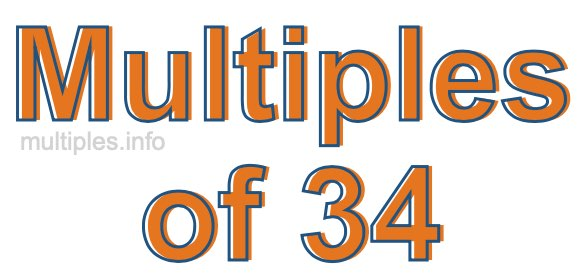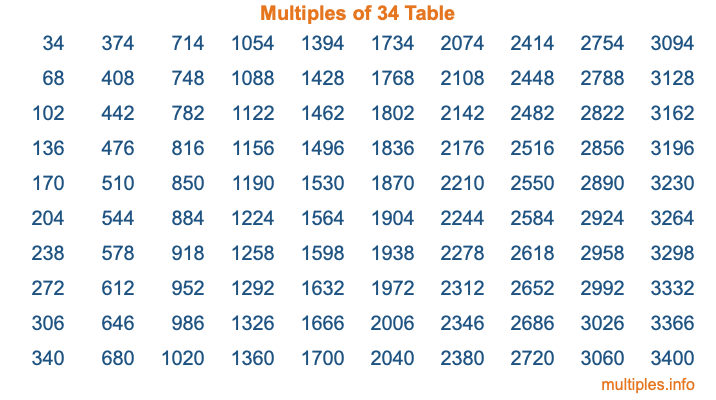Multiples of 34Welcome to the Multiples of 34 page. Here we will first teach you everything you will ever need to know about the multiples of 34, and then give you a study guide summary of everything we taught you to make sure you remember it all. Use this page to look up facts and learn information about the multiples of 34. This page will make you a multiples of thirty-four expert!

Definition of Multiples of 34
Multiples of 34 are all the numbers that when divided by 34 equal an integer. Each of the multiples of 34 are called a multiple. A multiple of 34 is created by multiplying 34 by an integer.

Therefore, to create a list of multiples of 34, you start with 1 multiplied by 34, then 2 multiplied by 34, then 3 multiplied by 34, and so on for as long as you want. Thus, the list of the first five multiples of 34 is 34, 68, 102, 136, and 170. To see a larger list of multiples of 34, see the printable image of Multiples of 34 further down on this page. We also have a category where you can choose any nth multiple of 34.

Multiples of 34 Checker
The Multiples of 34 Checker below checks to see if any number of your choice is a multiple of 34. In other words, it checks to see if there is any number (integer) that when multiplied by 34 will equal your number. To do that, we divide your number by 34. If the the quotient is an integer, then your number is a multiple of 34.

Is  a multiple of 34?

Least Common Multiple of 34 and ...
A Least Common Multiple (LCM) is the lowest multiple that two or more numbers have in common. This is also called the smallest common multiple or lowest common multiple and is useful to know when you are adding our subtracting fractions. Enter one or more numbers below (34 is already entered) to find the LCM.

Check out our LCM Calculator if you need more details about the Least Common Multiple or if you need the LCM for different numbers for adding and subtraction fractions.

nth Multiple of 34
As we stated above, 34 is the first multiple of 34, 68 is the second multiple of 34, 102 is the third multiple of 34, and so on. Enter a number below to find the nth multiple of 34.

th multiple of 34

Multiples of 34 vs Factors of 34
34 is a multiple of 34 and a factor of 34, but that is where the similarities end. All postive multiples of 34 are 34 or greater than 34. All positive factors of 34 are 34 or less than 34.

Below is the beginning list of multiples of 34 and the factors of 34 so you can compare:

Multiples of 34: 34, 68, 102, 136, 170, etc.

Factors of 34: 1, 2, 17, 34

As you can see, the multiples of 34 are all the numbers that you can divide by 34 to get a whole number. The factors of 34, on the other hand, are all the whole numbers that you can multiply by another whole number to get 34.

It's also interesting to note that if a number (x) is a factor of 34, then 34 will also be a multiple of that number (x).

Multiples of 34 vs Divisors of 34
The divisors of 34 are all the integers that 34 can be divided by evenly. Below is a list of the divisors of 34.

Divisors of 34: 1, 2, 17, 34

The interesting thing to note here is that if you take any multiple of 34 and divide it by a divisor of 34, you will see that the quotient is an integer.

Multiples of 34 Table
Below is an image of the first 100 multiples of 34 in a table. The table is in chronological order, column by column. The first column has the first ten multiples of 34, the second column has the next ten multiples of 34, and so on.The Multiples of 34 Table is also referred to as the 34 Times Table or Times Table of 34. You are welcome to print out our table for your studies.

Negative Multiples of 34
Although not often discussed or needed in math, it is worth mentioning that you can make a list of negative multiples of 34 by multiplying 34 by -1, then by -2, then by -3, and so on, to get the following list of negative multiples of 34:

-34, -68, -102, -136, -170, etc.

Multiples of 34 Summary
Below is a summary of important Multiples of 34 facts that we have discussed on this page. To retain the knowledge on this page, we recommend that you read through the summary and explain to yourself or a study partner why they hold true.

There are an infinite number of multiples of 34.

A multiple of 34 divided by 34 will equal a whole number.

34 divided by a factor of 34 equals a divisor of 34.

The nth multiple of 34 is n times 34.

The largest factor of 34 is equal to the first positive multiple of 34.

34 is a multiple of every factor of 34.

34 is a multiple of 34.

A multiple of 34 divided by a divisor of 34 equals an integer.

34 divided by a divisor of 34 equals a factor of 34.

Any integer times 34 will equal a multiple of 34.

Multiples of a Number
Here you can get the multiples of another number, all with the same attention to detail as we did for multiples of 34 on this page.

Multiples of
Multiples of 35
Did you find our page about multiples of thirty-four educational? Do you want more knowledge? Check out the multiples of the next number on our list!